#### You may also like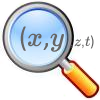### The Best Square

How would you judge a competition to draw a freehand square?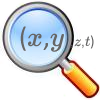### Trig-trig

Explore the properties of combinations of trig functions in this open investigation.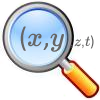### A Different Differential Equation

Explore the properties of this different sort of differential equation.

# Chance of That

##### Age 16 to 18Challenge Level

Yaseen from LAE Tottenham in the UK visualised the lists of numbers and used computer programming to generate pairs of lists whose correlation was exactly zero. This is Yaseen's work: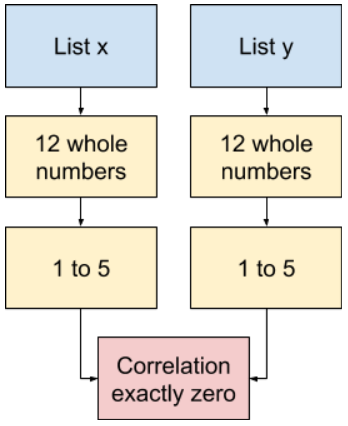I tried manually creating two seemingly random lists adhering to the parameters given, however, I had no luck. The value of the Pearson product-moment correlation coefficient (PPMCC) never hit exactly 0. Therefore, I used my Python skills to create a program that would generate the two lists for me with zero correlation.

The way it works:

1. Generate two random lists
1. 12 whole numbers
2. Each number between 1 and 5 inclusive
2. Calculate the correlation between the two lists.
1. This is done by importing a function that calculates r.
3. Check if r = 0
1. If it does, exit the loop.
2. If it does not, go back to step 1.
4. Output the two lists

Click below to see examples of lists generated by Yaseen's program.

 List x List y Graph 3, 2, 1, 1, 4, 3, 4, 2, 4, 4, 3, 5 2, 5, 1, 1, 4, 2, 2, 5, 5, 1, 4, 1 Figure 1 4, 2, 1, 4, 4, 2, 1, 1, 1, 2, 3, 3 3, 5, 3, 2, 2, 1, 1, 2, 5, 1, 4, 4 Figure 2 2, 1, 4, 3, 3, 3, 4, 2, 3, 2, 4, 1 3, 1, 3, 2, 1, 4, 2, 2, 3, 3, 4, 5 Figure 3 3, 4, 4, 3, 3, 1, 2, 2, 4, 2, 5, 3 3, 1, 5, 2, 4, 1, 2, 2, 1, 5, 2, 5 Figure 4 5, 2, 3, 4, 3, 3, 3, 2, 1, 5, 3, 2 5, 5, 5, 4, 4, 4, 3, 3, 5, 4, 2, 4 Figure 5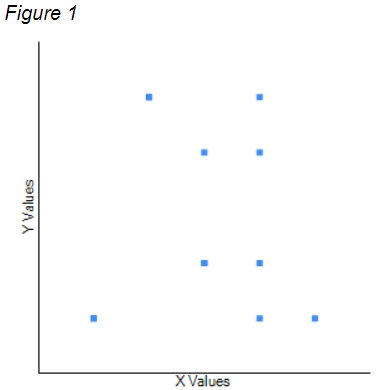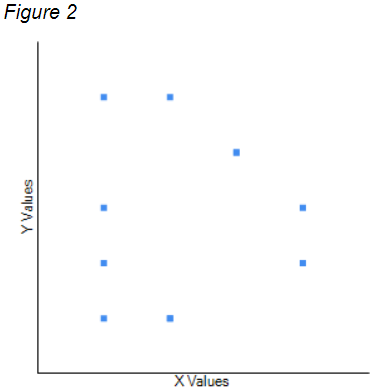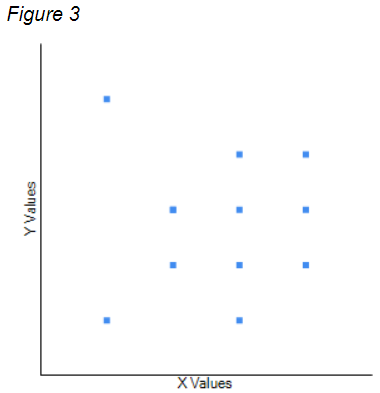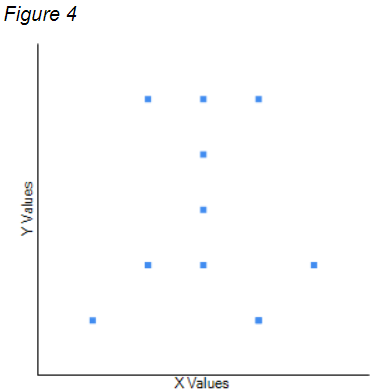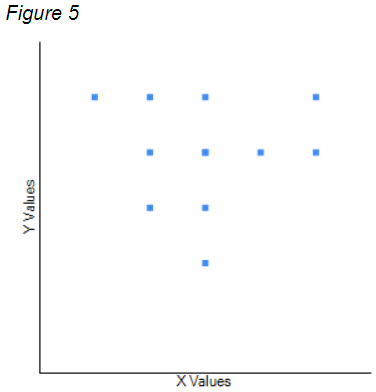Analysis

When I saw the graphs I was confused as there were [fewer] than twelve data points on each. I quickly realised that this is due to there being more than one data point at [the same] point on the graph. This meant that the graph was slightly misleading as acquiring a proper understanding of the graph requires knowledge of the raw data.

Yaseen also thought about patterns which might be present in the underlying numbers. Can you use these ideas to manually generate lists of numbers whose correlation coefficient is equal to zero?
Pearson's r has the following equation:

$r=\frac{\Sigma(x-\bar{x})(y-\bar y)}{\sqrt{(x-\bar x)^2(y-\bar y)^2}}$

A shorter representation:

$r=\dfrac{S_{xy}}{S_xS_y}$

When there is no correlation:

$r=0 \Rightarrow S_{xy}=0$

$\therefore\Sigma(x-\bar x)(y-\bar y)=0$

While I am not sure how to fully interpret this, I conjecture that there is some sort of cancelling occurring within the set of $(x-\bar x)(y-\bar y)$ values. It could consist of identical values with different signs (e.g. $-3,$ $-2,$ $-1,$ $+1,$ $+2,$ $+3$) or varying values that altogether sum to $0$ (e.g. $-10$, $-8$, $-4$, $-3$, $-1$, $+3$, $+5$, $+6$, $+12$). I feel the key to this lies within the product of the deviation scores.

Yaseen also imposed some constraints to his program to see whether it could still find lists with correlation coefficient equal to zero. Can you find a pair of lists manually which satisfies Yaseen's constraints? Two minutes might not have been long enough for the program to find lists which work.
I edited my Python code to generate two lists with zero correlation without the number $1$ present in either of the lists. I left the program running for over $2$ minutes yet no results were outputted. After this, I ran another experiment setting the condition that the number $1$ cannot be present in list $x$ and the number $2$ cannot be present in list $y.$ This also produced no results. This implies that two lists, following the given parameters, cannot exist with at least one number from $1$ to $5$ inclusive not being present in both lists or one number missing from list $x$ and another missing from list $y.$

With real data, you would not expect to get a value of $r$ of exactly $0.$ Even the slightest shift of a data point towards the line of best fit would change the value of $r.$